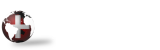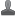####Author Topic: Resize pictures for Excel  (Read 492 times)

#### dalem

• New Member
•• Posts: 4##### Resize pictures for Excel
« on: September 25, 2019, 12:14:40 PM »
I've got a report that creates an excel spreadsheet. Each row data for an inventory item, with on cell being an image.
Of course, no 2 images are the same size.
I load the image into the cell:
31095       let ACTIVE_SHEET=EXCEL_OBJ'ACTIVESHEET
31100       let POS\$="C"+str(EXCEL_ROW)
31105       EXCEL_OBJ'RANGE(POS\$)'SELECT() ! Specific current cell
31110       let PICTURE\$=%_IMAGEDIR\$+IMAGE\$
31115       let PICTURES=ACTIVE_SHEET'PICTURES()
31120       PICTURES'INSERT(PICTURE\$)
----
Looking at a VB script, when I resize an image to .25 inches:
ActiveSheet.Shapes.Range(Array("Picture 3")).Select
Selection.ShapeRange.Height = 18
ActiveSheet.Shapes.Range(Array("Picture 4")).Select
Selection.ShapeRange.Height = 18

So, apparently each pic has a 'name'. Lucky, I kept count, and tried this:
32052>      for X=1 to IMAGES_FOUND
32053       let IMAGE_NAME\$="Picture "+str(X)
32060       let ACTIVE_SHEET=EXCEL_OBJ'ACTIVESHEET;
:           let SHAPES=ACTIVE_SHEET'SHAPES;
:           let RANGES=SHAPES'RANGE(ARRAY(IMAGE_NAME\$));
:           let SELECTION=RANGES'SELECT
32061       let SELECTION.SHAPERANGE.HEIGHT=18
32062       next X

But the "Let Ranges" is giving me an error 88.

Any ideas? Is there a better way?